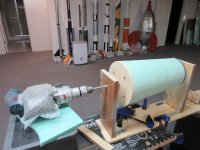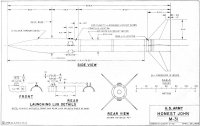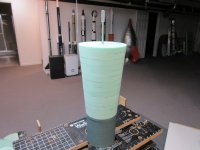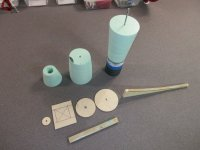# Need Math Help- 6" Dia. Honest John Nosecone### Help Support The Rocketry Forum:#### jmmome

##### Well-Known Member
TRF Supporter
May have inhaled too much foam board dust constructing the bottom 14" section of the nosecone. That part was (relatively) easy.

Now I need to construct the top 24" of nosecone, which is a 3:1 ogive. The base of it will be 8" in diameter to match the top of the bottom section I've already created.

So- 24" length for the centerline, and 4" for the radius............how do I calculate my pivot point length & location to create the ogive shape? I throw myself at the mercy of the court.

Thanks!!!!#### Brent

##### Well-Known Member
May have inhaled too much foam board dust constructing the bottom 14" section of the nosecone. That part was (relatively) easy.

Now I need to construct the top 24" of nosecone, which is a 3:1 ogive. The base of it will be 8" in diameter to match the top of the bottom section I've already created.

So- 24" length for the centerline, and 4" for the radius............how do I calculate my pivot point length & location to create the ogive shape? I throw myself at the mercy of the court.

Thanks!!!!

View attachment 440923View attachment 440924

##### Well-Known Member
I can't answer your question but just wanted to say when I first read the title of your thread, I thought you needed help with a dishonest john. I had to re-read it and then chuckled to myself a few times.

•jmmome

#### ThirstyBarbarian

##### Well-Known Member
TRF Supporter
I’m not sure how you would do it mathematically. When I did my foam project, I made an Open Rocket file, specified the nosecone type, and then printed out the template at full scale. It took a few sheets of paper that I had to tape together for the full template.

•K'Tesh and Dwatkins

#### boatgeek

##### Well-Known Member
May have inhaled too much foam board dust constructing the bottom 14" section of the nosecone. That part was (relatively) easy.

Now I need to construct the top 24" of nosecone, which is a 3:1 ogive. The base of it will be 8" in diameter to match the top of the bottom section I've already created.

So- 24" length for the centerline, and 4" for the radius............how do I calculate my pivot point length & location to create the ogive shape? I throw myself at the mercy of the court.

Thanks!!!!

View attachment 440923View attachment 440924
I don't know how to do this mathematically, because I always let AutoCAD do the math.The center of the curve is 5'-10" (70") from the center of the base of the ogive section. In other words, the radius of the ogive section is 74".

#### jmmome

##### Well-Known Member
TRF Supporter
I don't know how to do this mathematically, because I always let AutoCAD do the math.The center of the curve is 5'-10" (70") from the center of the base of the ogive section. In other words, the radius of the ogive section is 74".
Thanks!

#### K'Tesh

##### OpenRocket Chuck Norris
TRF Supporter
If you're looking for some HoJo nosecones sims I have a couple of them (as part of full sims) over in my OpenRocket Files thread.You can scale them up to your necessary diameter, and try something like Thirsty suggested. FWIW, I recently scored a set of original for the BT-55 Hojo (1919). I'm hoping that they'll be sent soon so I can try to scan everything accurately (in a way that I can see easily and clearly) for the 1919 sim. I've been sent decals for other Hojo's but I want to make sure that the 1919's sim matches the kit.

#### kuririn

##### BARGeezer
TRF Supporter
You have the drill/lathe.
You have the dimensional drawing.
Create a template by upscaling the drawing to your pieces.
Cut out the template on cardboard and turn your nose cone.
No math needed (except for simple ratios for the upscale), no equations to deal with.

•stantonjtroy

#### dr wogz

##### Fly caster
+1 on allowing Autocad do the math & print out a template

(Come to think of it.. I don't really remember the last time I did "math" [except build & tolerance stacks].. I always draw in ACAD, so it does my trig for me. FEM in Creo.. now that's another story!)

#### jmmome

##### Well-Known Member
TRF Supporter
I don't know how to do this mathematically, because I always let AutoCAD do the math.The center of the curve is 5'-10" (70") from the center of the base of the ogive section. In other words, the radius of the ogive section is 74".
The 74" dimension worked perfectly for drawing the template- thanks!

Not only will it help me to cut each 1" thick foam piece a little larger, but I'll also transfer the template to 1/2" plywood, cut two of them and glue together, epoxy sandpaper to the curve of the template, and see if it sands down the foam board as well as my first try with a straight 16" sanding board.

#### Nytrunner

##### Pop lugs, not drugs
TRF Supporter
Oh c'mon folks, this isn't the Barrowman equations, do the math and keep your brain from turning into mush!

Circles are annoying, do triangles instead!

Example, a circles is such:
$$r^2=(x-h)^2+(y-k)^2$$

Where [x,y] is the coordinates of your points on the circle and [h,k] are the coordinates of your center.
Since the radius is the same, you could write the circle equation for both points, set them equal to each other and solve for r and k (the radius, and the offset of the center)

If your center is at [0,0] then you end up with
$$x^2+y^2=r^2$$
which is suspiciously similar to the pythagorean theorem (triangle!)

You know 2 points [0,4] and [24,0] both of which are points along the circle that forms your ogive, and thus have the same radius.
Knowing that your circle center is at 0 on the x-axis simplifies things and allows us to skip the circle equation. As with any geometry problem, draw a picture first (exercise left to student)

Treating the cone axis as the x-axis, and cone base as the y-axis, we know your radius extends from circle center along the y-axis to your first point which is 4" above the x-axis [0,4]
If the first point [0,4] is above the x-axis, then your circle center will be below it and k is negative (sign convention is important!)

That means your radius is equal to 4 plus -k (the circle's y-offset)

$$(1): r=4+(-k)$$

If the 2nd point is on the x-axis (y=0) then we can draw a triangle with one leg equal to the center offset (-k) and the other leg equal to it's x position (24")

Applying pythagoras:
$$(2): r^2=24^2+(-k)^2$$
We once again have 2 equations with two variables: vertical circle offset (k) and circle radius (r) which you really want

Draw out -k from equation 1
$$(3): -k=r-4$$
Substitute in equation 2 so the whole thing is in terms of r
$$(4): r^2=24^2+(r-4)^2$$
Solving for r
$$0=576+r^2-8r+16-r^2=>$$
$$8r=592=>$$
$$r=74$$
You have the number you want, but it's best for to finish the calc and check yourself. If the radius is 74 then the circle offset can be found

$$74=-k+4=>$$
$$k=-70$$
That result matches our earlier statement that k would be a negative value reflecting the circle's position below the x-axis
Final check

$$74^2=24^2+(-70)^2=>$$
$$5476=576+4900=>$$
$$5476=5476$$

And if you plug those values into the circle equation at the top for both your coordinates, it'll check out there too

#### boatgeek

##### Well-Known Member
+1 on allowing Autocad do the math & print out a template

(Come to think of it.. I don't really remember the last time I did "math" [except build & tolerance stacks].. I always draw in ACAD, so it does my trig for me. FEM in Creo.. now that's another story!)
I always tell high school students interested in engineering that you need higher math to get an engineering degree, but you don't need higher math to do engineering. I rarely go past a square root, though I sometimes get into trig.

Oh c'mon folks, this isn't the Barrowman equations, do the math and keep your brain from turning into mush!

Circles are annoying, do triangles instead!

Example, a circles is such:
$$r^2=(x-h)^2+(y-k)^2$$

Where [x,y] is the coordinates of your points on the circle and [h,k] are the coordinates of your center.
Since the radius is the same, you could write the circle equation for both points, set them equal to each other and solve for r and k (the radius, and the offset of the center)

If your center is at [0,0] then you end up with
$$x^2+y^2=r^2$$
which is suspiciously similar to the pythagorean theorem (triangle!)

You know 2 points [0,4] and [24,0] both of which are points along the circle that forms your ogive, and thus have the same radius.
Knowing that your circle center is at 0 on the x-axis simplifies things and allows us to skip the circle equation. As with any geometry problem, draw a picture first (exercise left to student)

Treating the cone axis as the x-axis, and cone base as the y-axis, we know your radius extends from circle center along the y-axis to your first point which is 4" above the x-axis [0,4]
If the first point [0,4] is above the x-axis, then your circle center will be below it and k is negative (sign convention is important!)

That means your radius is equal to 4 plus -k (the circle's y-offset)

$$(1): r=4+(-k)$$

If the 2nd point is on the x-axis (y=0) then we can draw a triangle with one leg equal to the center offset (-k) and the other leg equal to it's x position (24")

Applying pythagoras:
$$(2): r^2=24^2+(-k)^2$$
We once again have 2 equations with two variables: vertical circle offset (k) and circle radius (r) which you really want

Draw out -k from equation 1
$$(3): -k=r-4$$
Substitute in equation 2 so the whole thing is in terms of r
$$(4): r^2=24^2+(r-4)^2$$
Solving for r
$$0=576+r^2-8r+16-r^2=>$$
$$8r=592=>$$
$$r=74$$
You have the number you want, but it's best for to finish the calc and check yourself. If the radius is 74 then the circle offset can be found

$$74=-k+4=>$$
$$k=-70$$
That result matches our earlier statement that k would be a negative value reflecting the circle's position below the x-axis
Final check

$$74^2=24^2+(-70)^2=>$$
$$5476=576+4900=>$$
$$5476=5476$$

And if you plug those values into the circle equation at the top for both your coordinates, it'll check out there too
On the other hand, it took me about 45 seconds to do the layout in CAD...#### Nytrunner

##### Pop lugs, not drugs
TRF Supporter
On the other hand, it took me about 45 seconds to do the layout in CAD...
It's your brain mush!•g.pitts and boatgeek

#### dr wogz

##### Fly caster
it's one thing to have the tool, it's entirely different thing to know how to use the tool!#### jmmome

##### Well-Known Member
TRF Supporter
Oh c'mon folks, this isn't the Barrowman equations, do the math and keep your brain from turning into mush!

Circles are annoying, do triangles instead!

Example, a circles is such:
$$r^2=(x-h)^2+(y-k)^2$$

Where [x,y] is the coordinates of your points on the circle and [h,k] are the coordinates of your center.
Since the radius is the same, you could write the circle equation for both points, set them equal to each other and solve for r and k (the radius, and the offset of the center)

If your center is at [0,0] then you end up with
$$x^2+y^2=r^2$$
which is suspiciously similar to the pythagorean theorem (triangle!)

You know 2 points [0,4] and [24,0] both of which are points along the circle that forms your ogive, and thus have the same radius.
Knowing that your circle center is at 0 on the x-axis simplifies things and allows us to skip the circle equation. As with any geometry problem, draw a picture first (exercise left to student)

Treating the cone axis as the x-axis, and cone base as the y-axis, we know your radius extends from circle center along the y-axis to your first point which is 4" above the x-axis [0,4]
If the first point [0,4] is above the x-axis, then your circle center will be below it and k is negative (sign convention is important!)

That means your radius is equal to 4 plus -k (the circle's y-offset)

$$(1): r=4+(-k)$$

If the 2nd point is on the x-axis (y=0) then we can draw a triangle with one leg equal to the center offset (-k) and the other leg equal to it's x position (24")

Applying pythagoras:
$$(2): r^2=24^2+(-k)^2$$
We once again have 2 equations with two variables: vertical circle offset (k) and circle radius (r) which you really want

Draw out -k from equation 1
$$(3): -k=r-4$$
Substitute in equation 2 so the whole thing is in terms of r
$$(4): r^2=24^2+(r-4)^2$$
Solving for r
$$0=576+r^2-8r+16-r^2=>$$
$$8r=592=>$$
$$r=74$$
You have the number you want, but it's best for to finish the calc and check yourself. If the radius is 74 then the circle offset can be found

$$74=-k+4=>$$
$$k=-70$$
That result matches our earlier statement that k would be a negative value reflecting the circle's position below the x-axis
Final check

$$74^2=24^2+(-70)^2=>$$
$$5476=576+4900=>$$
$$5476=5476$$

And if you plug those values into the circle equation at the top for both your coordinates, it'll check out there too
And to think i used to teach that stuff in high school in 1976 & 1977............your equations brought back memories of blank faces on kids in my class.

•g.pitts and Nytrunner

#### RocketRev

Uh....... blank faces..........on the kids..............yes..............that's what I was going to say too..........

Sorry, I'm laughing to hard!

•jmmome

#### itsmeGriff

##### Member
I can't answer your question but just wanted to say when I first read the title of your thread, I thought you needed help with a dishonest john. I had to re-read it and then chuckled to myself a few times.
Baahahaa, some brightness to my crappy day!

•jmmome

#### Ez2cDave

##### Well-Known Member
TRF Supporter
I don't know how to do this mathematically, because I always let AutoCAD do the math.The center of the curve is 5'-10" (70") from the center of the base of the ogive section. In other words, the radius of the ogive section is 74".
Time to go "Old School" . . . LOL !

See PDF's below.

Dave F.

#### Attachments

• 109.9 KB Views: 13
• 4.7 MB Views: 10
•jmmome

#### jmmome

##### Well-Known Member
TRF Supporter
So far- so good. First 14" of 6" body tube dia. to 8" fattest part of the nosecone done, including adding the 6" long shoulder which will go into the body tube.Next 8" of disks of the final 24" of the ogive portion are cut. I'll cut a 24" long ogive template, attach sandpaper to it's edge, and see if I can hinge it to the tip of the nosecone end of the sanding lathe. I'll start sanding from the 8" diameter, and work my way down to the tip by adding consecutively smaller foam board disks. I could "freehand" the ogive sanding, but I'd like to try this first.

#### Ez2cDave

##### Well-Known Member
TRF Supporter
HOJO . . .•jmmome

#### Cape Byron

##### The BAR formerly known as Skippy-2
TRF Supporter
Example, a circles is such:

r2=(x−h)2+(y−k)2​
Yep, and that's where I got totally lost. How about Skippy+Maths=Friends

#### Nytrunner

##### Pop lugs, not drugs
TRF Supporter
Yep, and that's where I got totally lost. How about Skippy+Maths=Friends
Rocketeers that don't like math? Scary!#### Cape Byron

##### The BAR formerly known as Skippy-2
TRF Supporter
Rocketeers that don't like math? Scary!I like maths fine, but it doesn't like me. I had to cram maths with a tutor to get into Uni as a mature age student studying Physics and Chemistry (and some other stuff). I have three university degrees, but maths I still struggle with. Use it or lose it, as they say.

TRF Supporter

#### Attachments

• 83.9 KB Views: 3

#### Nytrunner

##### Pop lugs, not drugs
TRF Supporter
Rocketeers have become dependent on "plugging everything into" a program or an app . . .
I wholly support the use of those tools (and use them 90% of the time). Once you know how to do the math, the tools let you do it more efficiently and in better time (and better understand the results and limitations).

#### Ez2cDave

##### Well-Known Member
TRF Supporter
I wholly support the use of those tools (and use them 90% of the time). Once you know how to do the math, the tools let you do it more efficiently and in better time (and better understand the results and limitations).
The "downfall of Modern Man" began with the invention of the "pocket calculator" and has progressed from there.

Dave F.

#### caveduck

##### semi old rocketeer
LOL I totally get the math but I bet you could look at the picture, turn that curve by hand and no one would be able to tell without a caliper#### hball55

##### Well-Known Member
it's one thing to have the tool, it's entirely different thing to know how to use the tool!You just described me perfectly#### jmmome

##### Well-Known Member
TRF Supporter
Wow- thanks to all!

Finished the nosecone- looks pretty close to what I was hoping for.

38" long. Fits a 6" Blue Tube. 8" (maybe a tad more) at its fattest diameter.

Still need to add the rest of the 3/8" threaded rod central spine, add the BB/epoxy nose weight between inches 6 to 12, and epoxy the four individual sections together. First photo shows some of the guides/stops I made to help keep the diameters consistent between the top of the previous section to the bottom of the next section. The ogive-shaped block & the straight block are what I attached 60 grit sandpaper to.